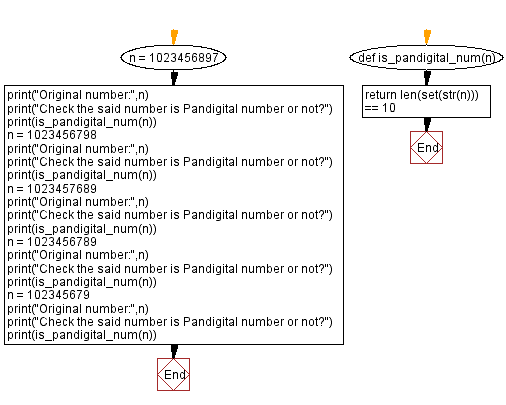﻿ Python: Test whether a given integer is pandigital number or not - w3resource# Python: Test whether a given integer is pandigital number or not

## Python Basic - 1: Exercise-106 with Solution

From Wikipedia,
In mathematics, a Pandigital number is an integer that in a given base has among its significant digits each digit used in the base at least once.
For example, 1223334444555556666667777777888888889999999990 is a Pandigital number in base 10. The first few Pandigital base 10 numbers are given by 1023456789, 1023456798, 1023456879, 1023456897, 1023456978, 1023456987, 1023457689.

Write a Python program to test whether a given integer is Pandigital number or not.

Sample Solution:

Python Code:

``````def is_pandigital_num(n):
return len(set(str(n))) == 10

n = 1023456897
print("Original number:",n)
print("Check the said number is Pandigital number or not?")
print(is_pandigital_num(n))
n = 1023456798
print("Original number:",n)
print("Check the said number is Pandigital number or not?")
print(is_pandigital_num(n))
n = 1023457689
print("Original number:",n)
print("Check the said number is Pandigital number or not?")
print(is_pandigital_num(n))
n = 1023456789
print("Original number:",n)
print("Check the said number is Pandigital number or not?")
print(is_pandigital_num(n))
n = 102345679
print("Original number:",n)
print("Check the said number is Pandigital number or not?")
print(is_pandigital_num(n))
``````

Sample Output:

```Original number: 1023456897
Check the said number is Pandigital number or not?
True
Original number: 1023456798
Check the said number is Pandigital number or not?
True
Original number: 1023457689
Check the said number is Pandigital number or not?
True
Original number: 1023456789
Check the said number is Pandigital number or not?
True
Original number: 102345679
Check the said number is Pandigital number or not?
False
```

Flowchart:Python Code Editor:

Have another way to solve this solution? Contribute your code (and comments) through Disqus.

What is the difficulty level of this exercise?

Test your Programming skills with w3resource's quiz.

﻿

## Python: Tips of the Day

What is the difference between Python's list methods append and extend?

append: Appends object at the end.

```x = [1, 2, 3]
x.append([4, 5])
print (x)
```

Output:

```[1, 2, 3, [4, 5]]
```

extend: Extends list by appending elements from the iterable.

```x = [1, 2, 3]
x.extend([4, 5])
print (x)
```

Output:

```[1, 2, 3, 4, 5]
```

Ref: https://bit.ly/2AZ6ZFq